# Describing Motion of a Rigid Body (Part - 2) Civil Engineering (CE) Notes | EduRev

## Civil Engineering (CE) : Describing Motion of a Rigid Body (Part - 2) Civil Engineering (CE) Notes | EduRev

The document Describing Motion of a Rigid Body (Part - 2) Civil Engineering (CE) Notes | EduRev is a part of the Civil Engineering (CE) Course Introduction to Dynamics and Vibrations- Notes, Videos, MCQs.
All you need of Civil Engineering (CE) at this link: Civil Engineering (CE)

6.2.2  Describing rotational motion: The angular velocity vector and spin tensor

We described the location of a particle in space using its position vector, and its motion using velocity.  We need to come up with something similar to velocity for rotations.

Definition of an angular velocity vector Visualize a spinning object, like the cube shown in the figure.  The box rotates about an axis – in the example, the axis is the line connecting two cube diagonals.  In addition, the object turns through some number of revolutions every minute.   We would specify the angular velocity of the shaft as a vector ω , with the following properties: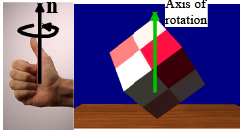1. The direction of the vector is parallel to the axis of the shaft (the axis of rotation). This direction would be specified by a unit vector n parallel to the shaft.

2. There are, of course, two possible directions for n.  By convention, we always choose a direction such that, when viewed in a direction parallel to n (so the vector points away from you) the shaft appears to rotate clockwise.  Or conversely, if n points towards you, the shaft appears to rotate counterclockwise. (This is the `right hand screw convention’)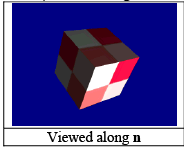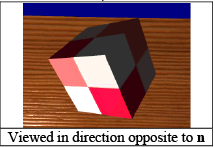3. The magnitude of the vector is the angular speed dθ / dt of the object, in radians per second.  If you know the revs per minute n turned by the shaft, the number of radians per sec follows as dθ / dt = 120πn .  The magnitude of the angular velocity is often denoted by ω = dθ / dt

The angular velocity vector is then  ω = dθ/dt n = ωn .

Since angular velocity is a vector, it has components ω = ωxi +ωyj+ ωzk in a fixed Cartesian basis.

As always, in two dimensions, everything is very simple.   In this case objects can only rotate about the k axis, and we can write the angular velocity vector as

ω = dθ/dt k

where θ is the counterclockwise angle of rotation of any line embedded in the body.

Writing down angular velocities:

For 2D problems, we always know the direction of the angular velocity and can just use ω = ωzk to write it down (of course if we know the value or a formula for ωz we can use it).

For 3D problems, we can often use vector addition to write down ω . We can illustrate this with a simple example:

Example: The propeller on the aircraft shown in the figure spins (about its axis) at 2000 rpm.   The aircraft travels at speed 200 km/hr in a turn with radius 1 km.    What is the angular velocity vector of (i) the body of the aircraft, and (ii) the propeller?  Express your answer in the normal-tangentialvertical basis.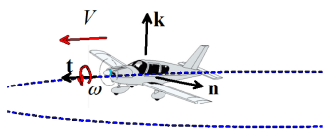(i) Note that:

• The circumference of the circle is s = 2πR = 2π km .
• The airplane completes a full circle in t = s / V = (2π / 200) × 3600 = 36π sec .
• A full turn is 2π radians, so the aircraft body turns at a rate 2π / (36π ) = (1/18)k rad/s about the k axis.

(ii) The propeller turns at 2000 rpm relative to the body of the plane. The angular velocity of the prop with respect to a stationary observer is therefore the vector sum of the 2000 rpm about the t axis, plus the angular velocity of the body. This gives

ωprop = [2000 x 2π/3600]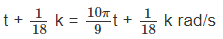Relation between the rotation matrix and the angular velocity vector: the spin tensor

We might guess that the angular velocity vector is the derivative of the rotation tensor. This is sort of correct, but the full story is a bit more complicated. The relationship between R and ω is constructed as follows:

1. We define the spin tensor W as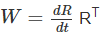2. The spin tensor is always skew ( W = −WT ), and we can read off the angular velocity vector by looking at its components.  Specifically, if ω = ωxi +ωyj+ ωzk then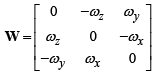We can use this formula in two ways: (1) Given R, we can calculate W and then read off the angular velocity vector components.  Alternatively, if we know ω , we can calculate R by first constructing W, then integrating the formula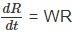Angular velocity-rotation relations in 2D

We can check this for the special case of a 2D rotation: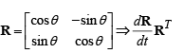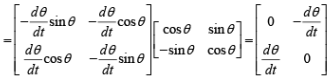As expected, we find that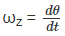This means that in 2D, angular velocity and the angle of rotation θ are related by the same formulas as distance traveled and speed for position. We can use all the same rules of calculus to go back and forth between them.

Angular velocity-Spin tensor formula

There is an important formula relating W and ω . Let rB −rA be a vector joining any two points in a rigid body. Then

W(rB − rA) = ω × (rB− rA)

You can see this by just multiplying out the definition of W and comparing the result to the cross product: if rB − rA = xi + yj +zk, then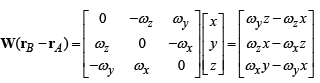Hopefully you can see that this is the same as the cross product!

6.2.3 The angular acceleration vector

Angular acceleration is the time derivative of angular velocity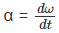For 3D, we can use

αx = dωx/dt     αy/dt   αz = dωz/dt

For 3D, we can’t express the angular accelerations or velocities as derivatives of rotation angles, because these can’t be defined for a general motion.

For a 2D problem, the direction of angular velocity and acceleration are known, so we have

α =αzk ω =ωzk

The components are related by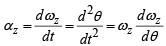For 2D problems, we can use all the usual rules of calculus to go from angular acceleration to angular velocity to angle, and vice-versa (just like distance-speed-acceleration formulas for straight line motion).

6.2.3 Relative velocity and acceleration of two points in a rigid body

We now know how to describe rotational motion. Our next order of business is to discuss a couple of very important formulas that we use to analyze the motion of a system of rigid bodies, and also to derive formulas for the angular momentum and kinetic energy of a rigid body..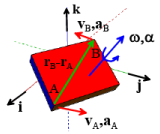Consider a rigid body:

Let ω be the (instantaneous) angular velocity of the body, and W the corresponding spin tensor Let A and B be two arbitrary points in a rigid body, and let rA ,rB and vA ,vB , aA , aB be their (instantaneous) position, velocity and acceleration vectors.

Then the relative position and velocity of A and B are related by

vB - vA = ω x (rB - rA )
vB - vA = W(rB - rA )

The relative acceleration of A and B are related their relative positions and velocity by

aB − aA = α×(rB−rA) + ω×(vB − vA) = α ×(rB−rA) + ω× [ω×(rB−rA)]

For 2D problems only: we can simplify these, because we know ω is always parallel to the k direction. Therefore

vB − vAzk × (rB − rA)

aB − aAzk × (rB −rA) −ωz2 (rB −rA)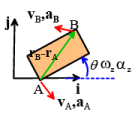Proof: These fornulas are easy to prove. Remember the mapping:

rB - rA = R(PB - PA ) ⇒ vB - vA = d/dt (rB-rA) = dR/dt(PB-PA)

Also,

rB − rA = R(pB −pA) ⇒ RT (rB − rA) = RT R(pB −pA) = (pB −pA)

Hence

vB - vA = dR/dt R(rB-rA)=W(rB-rA)

Remember that W(rB − rA) = ω × (rB− rA) , so the acceleration formula then follows as

aB-aA=d/dt(vB-vA)=dω/dt x(rB-rA)+ω x d/dt(rB-rA)=α x (rB-rA)+ω x (vB-vA)

Offer running on EduRev: Apply code STAYHOME200 to get INR 200 off on our premium plan EduRev Infinity!

## Introduction to Dynamics and Vibrations- Notes, Videos, MCQs

20 videos|53 docs

,

,

,

,

,

,

,

,

,

,

,

,

,

,

,

,

,

,

,

,

,

;